Want to share your content on R-bloggers? click here if you have a blog, or here if you don't.

## Code for fun

I feel passionately that anyone can learn some coding but many feel they lack natural aptitude for it.
One of the things I always try to stress to those I am teaching is that it matters very much less what you do than that do you it. It doesn’t matter how small and trivial the result of the code is, or even how much of it you wrote yourself. Any work you do with code at all, will build your confidence, experience and skills.

I recently wrote this post which included this R-generated plot: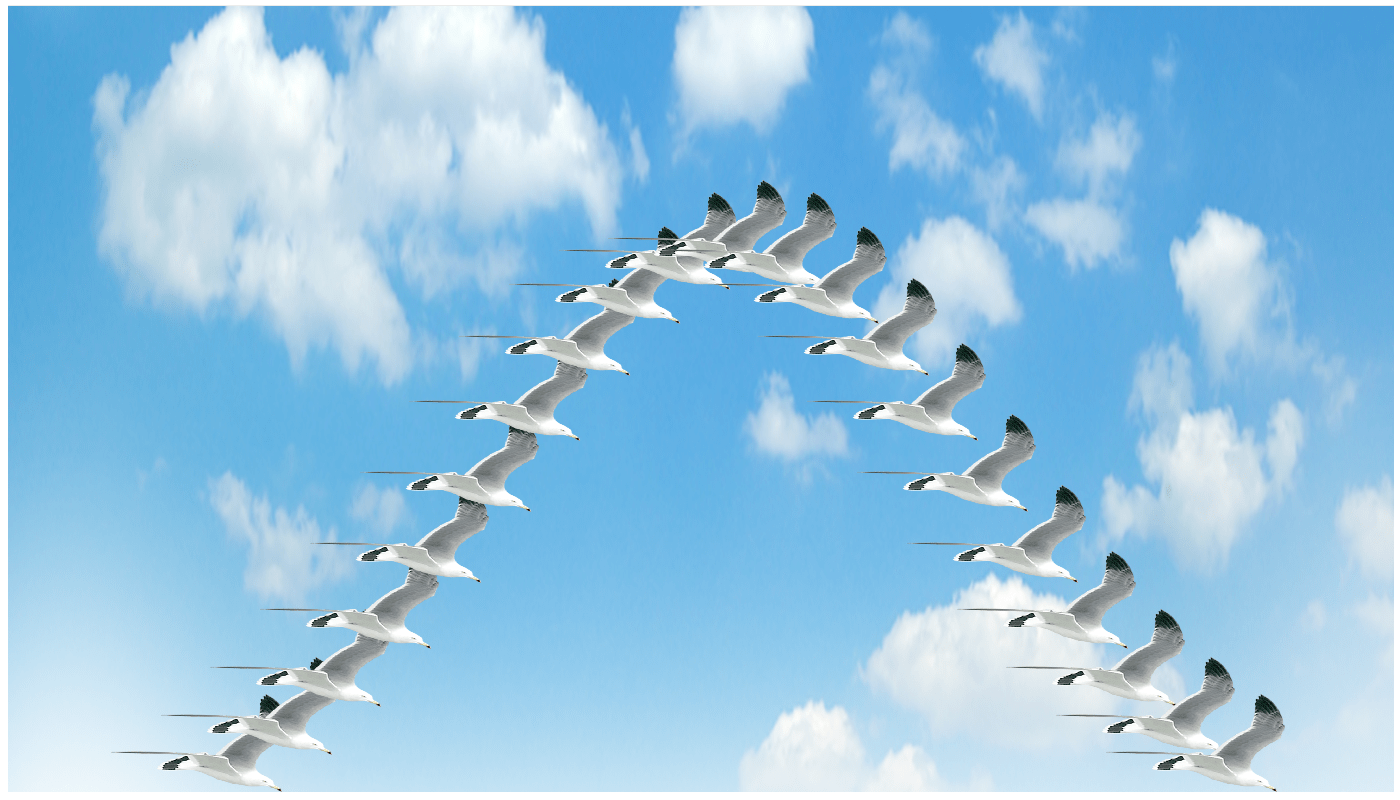Now I’m going to show you how to plot some data using one image as a marker and a different one for the back ground!

I will assume you have Rstudio installed and have at least a little experience with it but I’m aiming to make this do-able for beginners. I’ll also be using ggplot2 rather than base R.

### 1. Preparing

The first thing you want to do is make a folder on your computer in which the code you write and your images will live. Now you need some images.
I’m going to this as my backgroundand this as my marker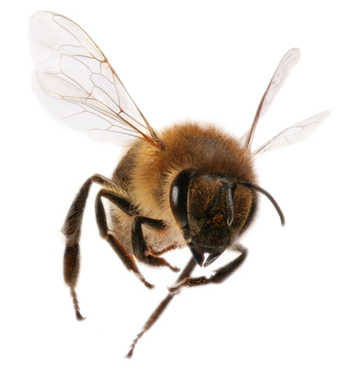You can use the same ones or use choose your own favourite images – try googling “public domain images”. You don’t want very high resolution images for the sake of speed but otherwise the size doesn’t matter.

The important thing is to save the images in to the folder you just created.

### 2. Getting started in RStudio

Start RStudio and set your working directory to the folder you created: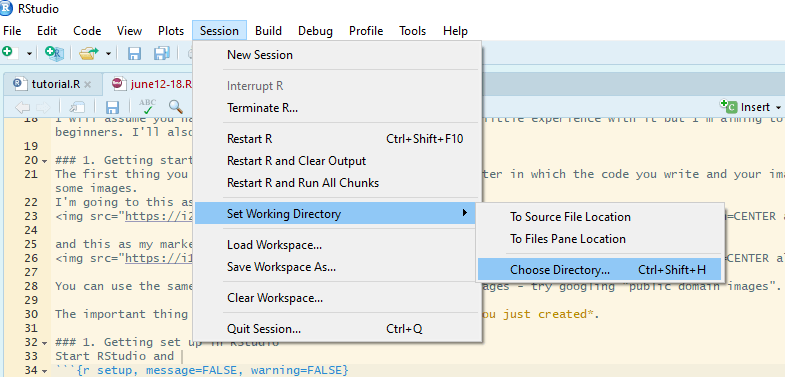Now start a new script file: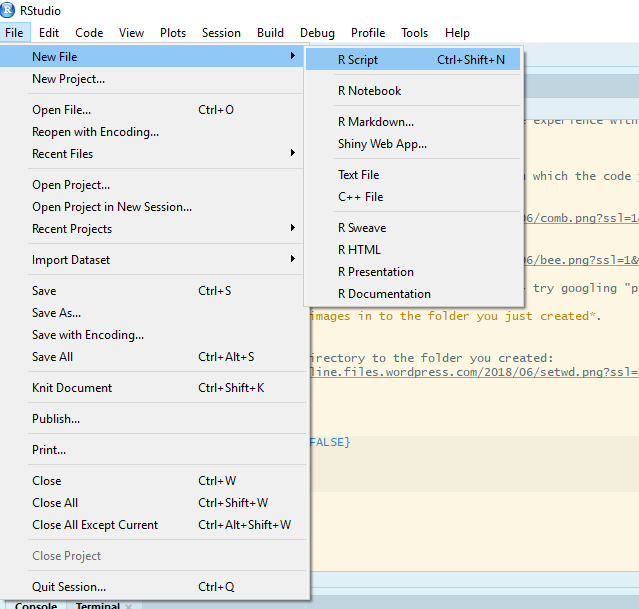and save it as funplot.R or similar.

There are several packages you will need. If your images files are png like mine: ggplot2, png, grid, ggimage.
If your images files are jpeg: ggplot2, jpeg, grid, ggimage.

All these are already installed for biologists at the University of York. If you are on your own computer you will need to install them.

Click the install button and write the package names in the box to install them. I recommending doing them one at a time.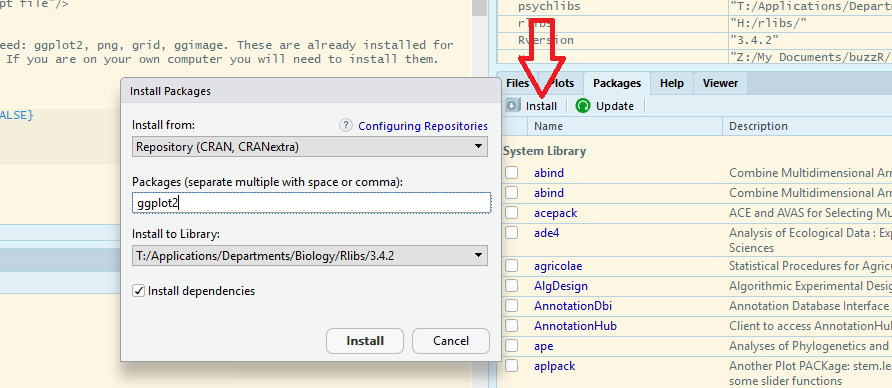Rstudio may need to install additional packages.

When everything is finished, you are ready to start coding!

### 3. Starting to code

Make the packages available for use in this Rstudio session by adding this code to your script and running it.

```library(ggplot2)
library(png)
library(grid)
library(ggimage)
```

### 4. Making some data to plot

I am going to plot the number of bees arriving within one hour at food sources placed at different distances from the hive. The data are as follows:

Distance (km) No.bees
0.5 40
1.0 34
1.5 31
2.0 22
2.5 18
3 10

To put these data in to a dataframe in R add this code to your script

```bees <- data.frame(distance = c(0.5, 1, 1.5, 2, 2.5, 3),
number = c(40, 34, 32, 22,18, 10))
```

Run that code. You do not need to re-run the first lines of code, just select the new lines and click run:### 5. A boring graph of the dataA simple default can be obtained with:

```ggplot(data = bees, aes(x = distance, y = number)) +
geom_point()
```Edit that code to add information about the axis labels and axis limits

```ggplot(data = bees, aes(x = distance, y = number)) +
geom_point() +
xlab("Distance (km)") +
ylab("Number of Bees") +
ylim(0, 45)
```### 6. A fun graph of the data!We need to read in the background file:

```img <- readPNG("comb.png")
```

Then we can edit the code with a `annotation_custom()` to add the background image:

```ggplot(data = bees, aes(x = distance, y = number)) +
annotation_custom(rasterGrob(img,
width = unit(1,"npc"),
height = unit(1,"npc")),
-Inf, Inf, -Inf, Inf) +
geom_point() +
xlab("Distance (km)") +
ylab("Number of Bees") +
ylim(0, 45)
```Make sure you put the `annotation_custom()` before the `geom_point()` but don’t worry too much about what the other bits mean.

To use bees as markers we need to add the bee image file name to every row of the data frame:

```bees\$image <- "bee.png"
```

This adds a column called image to the dataframe called bees. We don’t have to explicitly read the image in because the `ggimage` package we loaded takes care of that. `ggimage` is an ‘extension’ to ggplot2.

Now edit your graph code again by removing the `geom_point()` and adding a `geom_image()` instead like this:

```ggplot(data = bees, aes(x = distance, y = number)) +
annotation_custom(rasterGrob(img,
width = unit(1,"npc"),
height = unit(1,"npc")),
-Inf, Inf, -Inf, Inf) +
geom_image(aes(image = image), size = 0.15) +
xlab("Distance (km)") +
ylab("Number of Bees") +
ylim(0, 45)
```You can adjust the size of the marker by changing `size = 0.15` and get rid of the gray background (and add axes) with:

```ggplot(data = bees, aes(x = distance, y = number)) +
annotation_custom(rasterGrob(img,
width = unit(1,"npc"),
height = unit(1,"npc")),
-Inf, Inf, -Inf, Inf) +
geom_image(aes(image = image), size = 0.15) +
xlab("Distance (km)") +
ylab("Number of Bees") +
ylim(0, 45) +
theme(panel.background = element_rect(fill = "white"))+
theme(axis.line.x = element_line(color = "black"),
axis.line.y = element_line(color = "black"))
```Ta da!! Hope you enjoyed it and it was easy to follow.